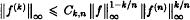Hostname: page-component-7d8f8d645b-dvxft Total loading time: 0 Render date: 2023-05-27T10:13:02.203Z Has data issue: false Feature Flags: { "useRatesEcommerce": true } hasContentIssue false

# On an inequality of Kolmogorov and Stein

Published online by Cambridge University Press:  17 April 2009

## Abstract

HTML view is not available for this content. However, as you have access to this content, a full PDF is available via the ‘Save PDF’ action button.

A.N. Kolmogorov showed that, if f, f′, …, f (n) are bounded continuous functions on ℝ, thenwhen 0 < k < n. This result was extended by E.M. Stein to Lebesgue Lp-spaces and by H.H. Bang to Orlicz spaces. In this paper, the inequality is extended to more general function spaces.

Type
Research Article
Information
Bulletin of the Australian Mathematical Society , February 2000 , pp. 153 - 159

## References

#### REFERENCES

Bang, H.H., ‘A remark on the Kolomogorov–Stein inequality’, J. Math. Anal. Appl. 203 (1996), 861867.CrossRefGoogle Scholar
Bertrandias, J.P. and Dupusi, C., ‘Transformation de Fourier sur les espaces lp (Lp′)’, Ann. Inst. Fourier Grenoble 29 (1979), 189206.CrossRefGoogle Scholar
Bloom, W.R., ‘Estimates for the Fourier transform’, Math. Scientist 10 (1985), 6581.Google Scholar
Certain, M.W. and Kurtz, T.G., ‘Landau–Kolmogorov inequalities for semigroups and groups’, Proc. Amer. Math. Soc. 63 (1977), 226230.CrossRefGoogle Scholar
Fournier, J.J.F., ‘On the Hausdorff–Young theorem for amalgams’, Monatsh. Math. 95 (1983), 117135.CrossRefGoogle Scholar
Holland, F., ‘Harmonic analysis on amalgams of Lp and lq’, J. London Math. Soc. 10 (1975), 295305.CrossRefGoogle Scholar
Holland, F., ‘On the representation of functions as Fourier transforms of unbounded measures’, Proc. London Math. Soc. 30 (1975), 347365.CrossRefGoogle Scholar
Kolmogorov, A.N., ‘On inequalities between upper bounds of the successive derivatives of an arbitrary function on an infinite interval’, Uchen. Zap. Moskov. Gos. Uni. 30 (1939), 316.Google Scholar
Kwong, M.K. and Zettl, A., Norm inequalities for derivatives and differences, Lecture Notes in Math. 1536 (Springer-Verlag, Berlin, Heidelberg, New York, 1992).CrossRefGoogle Scholar
Stein, E.M., ‘Functions of exponential type’, Ann. Math. 65 (1957), 582592.CrossRefGoogle Scholar
Tikhomirov, V.M. and Magaril-II'jaev, G.G., ‘Inequalities for derivatives’, in Kolmogorov, A.N., Selected Papers (Nauka, Moscow, 1985).Google Scholar